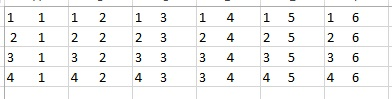# Probability: An Introduction

Many popular machine learning algorithms are based on probability. If you are a bit shaky on your probability, don’t worry this quick primer will get you up to speed.

Think about a coin flip. There are 2 possibilities you could have (heads or tails). So if you wanted to know the probability of getting heads in any particular flip, it would be 1/2 (desired outcome/all possible outcomes).

Now take a 6 sided die:

• The probability rolling a 1 is 1/6.
• rolling an even number (2,4,6) = 3/6 or 1/2
• rolling a number less than 3 (1,2) = 2/6 or 1/3

The compliment of a probability can also be referred to the probability of an event NOT happening. The probability of not rolling a 1 on a six sided die = 5/6.

P(~A) = 1 – P(A) = 1 – 1/6 = 5/6

## Independent Probability

Independent probability simply means determining the probability of 2 or more events when the outcome of one event has no effect on the other.

Let’s take two coins (A and B). Take the first coin and flip it. Imagine it comes up heads. Now flip the second coin. The fact that the first coin can up heads will not influence the outcome of the second flip in any way. To show this mathematically:

• Probability of flipping heads coin A = P(A) = 1/2
• Probability of flipping heads coin B = P(B) = 1/2
• Probability of flipping 2 heads = P(A and B) = P(A ∩ B) = P(A)*P(B)=1/2*1/2=1/4

## Mutually Exclusive

Now we are asking if event A or B occurred.

P(A or B) = P(A∪B) = P(A) +P(B)

So the probability of 10 or a 2 from a deck of cards = 1/52 + 1/52 = 2/52 = 1/26

## Not Mutually Exclusive

Imaging drawing an Ace and a Red Card. We want to make sure to factor in all the elements, but we need to account for double counting.

P(A or B) = P(A∪B) = P(A) +P(B) – P(A and B)

4/52 (ACE) + 26/52(Red Card) – 2/52(To get both an Ace and a Red card, the only options are Ace of Hearts and Ace of Diamonds) = 28/52 = 7/13

## Conditional Probability

Now we are going to work with dice. One six sided die and one 4 sided die. The diagram below shows all 24 possible combinations.Now conditional probability is the probability of something occurring assuming some prior event has occurred.  Look at the chart above, lets consider the A = rolling even number on six sided die (3/6) and B = rolling even number on 4 side die(2/4). So P(A|B) (read probability of A given B) = P(A∩B)/P(B). Lets look a the chart to help use see this.So, when rolling a six sided die (A), you have a 3/6 chance of rolling an even number(2,4,6)

When rolling a four sided die (B), you have a 2/4 chance of rolling an even number(2,4)

So P(A and B)  = 3/6*2/4=6/24=1/4

Now when figuring P(A|B) (rolling an even on the four side die assuming you have already rolled an even on the six sided die) we are no longer looking at all 24 combinations, we are now only looking at the combination where the six side die (A) is even (the green columns). So as you can see, of the 12 options where A is even, 6 have an even number on the 4 sided die.

So… P(A ∩ B)/P(B) = (1/4)/(1/2) = 1/2. Which makes sense since of the 12 combinations where A is even, 6 have even numbers for B. 6/12 = 1/2